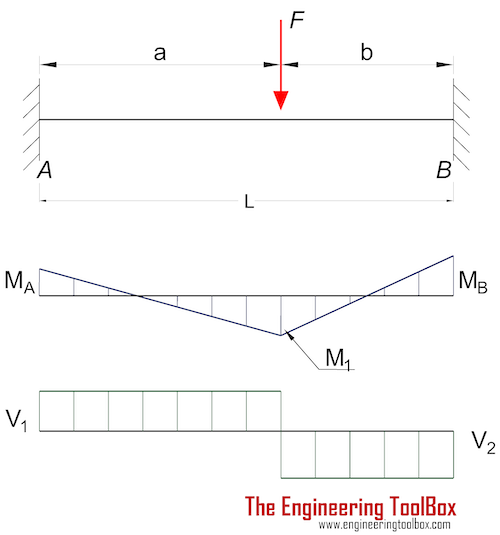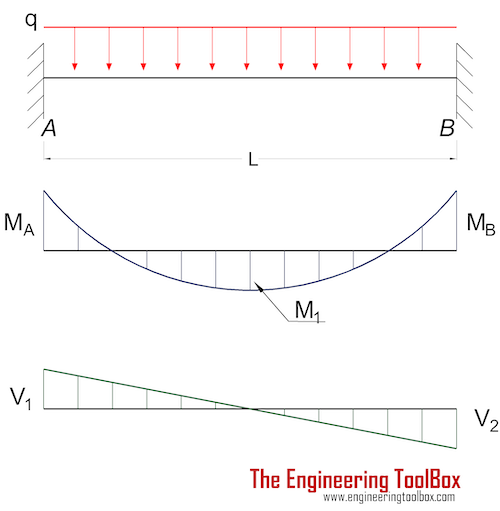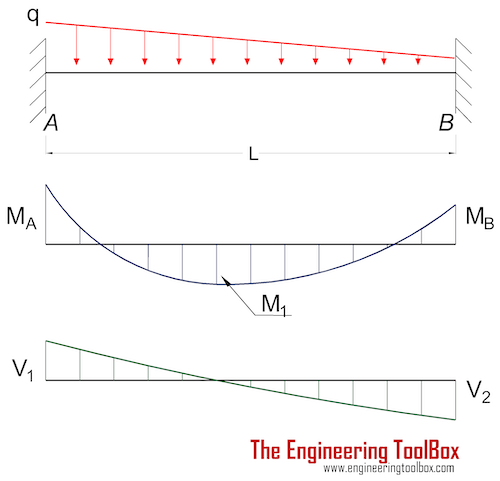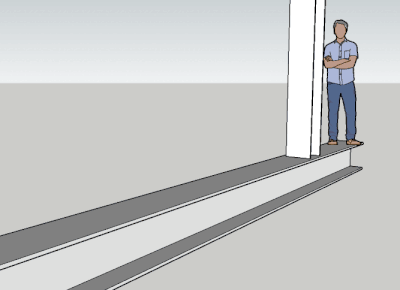Engineering ToolBox - Resources, Tools and Basic Information for Engineering and Design of Technical Applications!

# Beams - Fixed at Both Ends - Continuous and Point Loads

## Stress, deflections and supporting loads.

### Beam Fixed at Both Ends - Single Point Load

####Bending Moment

MA = - F a b2 / L2                               (1a)

where

MA = moment at the fixed end A (Nm, lbf ft)

MB = - F a2 b / L2                              (1b)

where

MB = moment at the fixed end B (Nm, lbf ft)

MF = 2 F a2 b2 / L3                           (1c)

where

MF = moment at the point load (Nm, lbf ft)

#### Deflection

δF = F a3 b3 / (3 L3 E I)                                  (1d)

where

δF = deflection at point load (m, ft)

E = Modulus of Elasticity (Pa (N/m2), N/mm2, psi)

I = Area Moment of Inertia (m4, mm4, in4)

#### Support Reactions

RA = F (3 a + b) b2 / L3                                 (1f)

where

RA = support force at fixed end A (N, lbf)

RB = F (a + 3 b) a2 / L3                                 (1g)

where

RB = support force at fixed end B  (N, lbf)

### Beam Fixed at Both Ends - Uniform Continuous Distributed Load

####Bending Moment

MA = MB

= - q L2 / 12                                   (2a)

where

M = moments at the fixed ends  (Nm, lbf ft)

q = uniform load (N/m, lbf/ft)

M1 = q L2 / 24                         (2b)

where

M1 = moment at the center (Nm, lbf ft)

#### Deflection

δmax = q L4 / (384 E I)                                  (2c)

where

δmax = max deflection at center (m, ft)

E = Modulus of Elasticity (Pa (N/m2), N/mm2, psi)

I = Area Moment of Inertia (m4, mm4, in4)

#### Support Reactions

RA = RB

=  q L / 2                               (2d)

where

R = support forces at the fixed ends  (N, lbf)

### Beam Fixed at Both Ends - Uniform Declining Distributed Load

####Bending Moment

MA = - q L2 / 20                                  (3a)

where

MA = moments at the fixed end A  (Nm, lbf ft)

q = uniform declining load (N/m, lbf/ft)

MB = - q L2 / 30                                 (3b)

where

MB = moments at the fixed end B  (Nm, lbf ft)

M1 = q L2 / 46.6                         (3c)

where

M1 = moment at x = 0.475 L (Nm, lbf ft)

#### Deflection

δmax = q L4 / (764 E I)                                  (3d)

where

δmax = max deflection at x = 0.475 L (m, ft)

E = Modulus of Elasticity (Pa (N/m2), N/mm2, psi)

I = Area Moment of Inertia (m4, mm4, in4)

δ1/2 = q L4 / (768 E I)                                  (3e)

where

δ1/2 = deflection at x = 0.5 L (m, ft)

#### Support Reactions

RA = 7 q L / 20                               (3f)

where

RA = support force at the fixed end A  (N, lbf)

RB =  3 q L / 20                               (3g)

where

RB = support force at the fixed end B  (N, lbf)

### Beam Fixed at Both Ends - Partly Uniform Continuous Distributed Load

####Bending Moment

MA = - (q a2 / 6) (3 - 4 a / l + 1.5 (a / L)2)                                  (4a)

where

MA = moment at the fixed end A  (Nm, lbf ft)

q = partly uniform load (N/m, lbf/ft)

MB = - (q a2 / 3) (a / L - 0.75 (a / L)2)                                 (4b)

where

MB = moment at the fixed end B  (Nm, lbf ft)

#### Support Reactions

RA = q a (L - 0.5 a) / L - (MA - MB) / L                              (4c)

where

RA = support force at the fixed end A  (N, lbf)

RB =  q a2 / (2 L) + (MA - MB) / L                              (4d)

where

RB = support force at the fixed end B  (N, lbf)

### Insert beams to your Sketchup model with the Engineering ToolBox Sketchup Extension## Related Topics

• ### Beams and Columns

Deflection and stress, moment of inertia, section modulus and technical information of beams and columns.
• ### Mechanics

Forces, acceleration, displacement, vectors, motion, momentum, energy of objects and more.
• ### Statics

Loads - forces and torque, beams and columns.

## Related Documents

• ### Aluminum I-Beams

Dimensions and static properties of aluminum I-beams - Imperial units.
• ### American Standard Beams - S Beam

American Standard Beams ASTM A6 - Imperial units.
• ### American Standard Steel C Channels.

Dimensions and static parameters of American Standard Steel C Channels
• ### American Wide Flange Beams

American Wide Flange Beams ASTM A6 in metric units.
• ### Area Moment of Inertia - Typical Cross Sections I

Typical cross sections and their Area Moment of Inertia.
• ### Area Moment of Inertia - Typical Cross Sections II

Area Moment of Inertia, Moment of Inertia for an Area or Second Moment of Area for typical cross section profiles.
• ### Area Moment of Inertia Converter

Convert between Area Moment of Inertia units.
• ### Beam Loads - Support Force Calculator

Calculate beam load and supporting forces.

• ### Beams Natural Vibration Frequency

Estimate structures natural vibration frequency.
• ### British Universal Columns and Beams

Properties of British Universal Steel Columns and Beams.
• ### Cantilever Beams - Moments and Deflections

Maximum reaction forces, deflections and moments - single and uniform loads.
• ### Continuous Beams - Moment and Reaction Support Forces

Moments and reaction support forces with distributed or point loads.
• ### Drawbridge - Force and Moment vs. Elevation

Calculate the acting forces and moments when elevating drawbridges or beams.
• ### Floor Joist Capacities

Carrying capacities of domestic timber floor joists - Grade C - metric units.
• ### Floors - Live Loads

Floors and minimum uniformly distributed live loads.
• ### HE-A Steel Beams

Properties of HE-A profiled steel beams.
• ### HE-B Steel Beams

Properties of HE-B profiled steel beams.
• ### HE-M Steel Beams

Properties of HE-M profile steel beams.
• ### Normal Flange I-Beams

Properties of normal flange I profile steel beams.
• ### Section Modulus - Unit Converter

Convert between Elastic Section Modulus units.
• ### Square Hollow Structural Sections - HSS

Weight, cross sectional area, moments of inertia - Imperial units
• ### Steel Angles - Equal Legs

Dimensions and static parameters of steel angles with equal legs - imperial units.
• ### Steel Angles - Equal Legs

Dimensions and static parameters of steel angles with equal legs - metric units.
• ### Steel Angles - Unequal Legs

Dimensions and static parameters of steel angles with unequal legs - imperial units.
• ### Steel Angles - Unequal Legs

Dimensions and static parameters of steel angles with unequal legs - metric units.
• ### Stiffness

Stiffness is resistance to deflection.
• ### Stress

Stress is force applied on cross-sectional area.
• ### Three-Hinged Arches - Continuous and Point Loads

Support reactions and bending moments.
• ### Trusses

Common types of trusses.

• ### W-Beams - American Wide Flange Beams

Dimensions of American Wide Flange Beams ASTM A6 (or W-Beams) - Imperial units.
• ### Weight of Beams - Stress and Strain

Stress and deformation of vertical beams due to own weight.

## Engineering ToolBox - SketchUp Extension - Online 3D modeling!

Add standard and customized parametric components - like flange beams, lumbers, piping, stairs and more - to your Sketchup model with the Engineering ToolBox - SketchUp Extension - enabled for use with older versions of the amazing SketchUp Make and the newer "up to date" SketchUp Pro . Add the Engineering ToolBox extension to your SketchUp Make/Pro from the Extension Warehouse !

We don't collect information from our users. More about

## Citation

• The Engineering ToolBox (2004). Beams - Fixed at Both Ends - Continuous and Point Loads . [online] Available at: https://www.engineeringtoolbox.com/beams-fixed-both-ends-support-loads-deflection-d_809.html [Accessed Day Month Year].

Modify the access date according your visit.

9.19.12